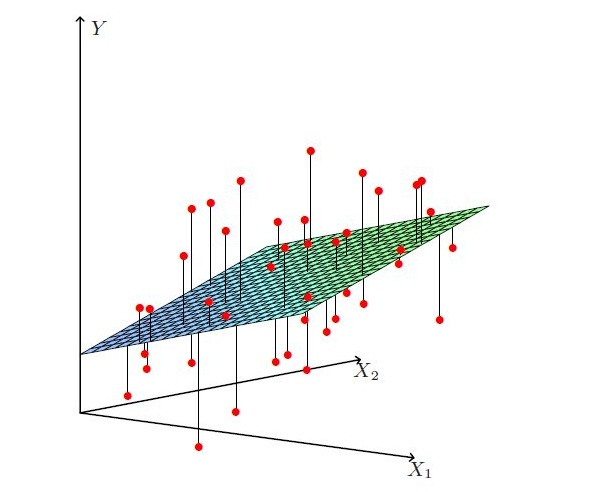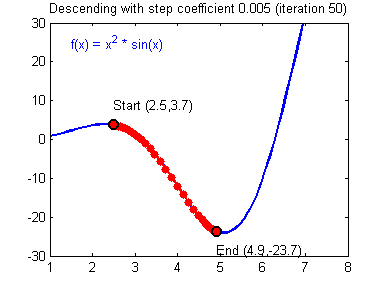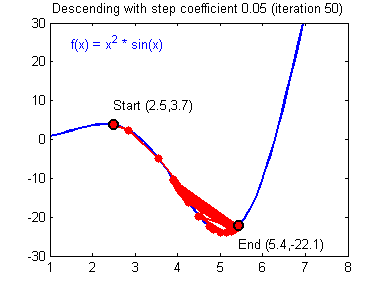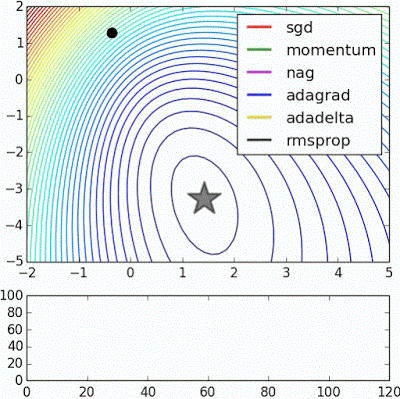# 线性回归的求解：矩阵方程和梯度下降、数学推导及NumPy实现

## Normal Equation​

### 一元线性回归​

$L(a, b) =\frac{1}{m} \sum_{i=1}^m[(ax^{(i)} + b) - y^{(i)}]^2 \tag{1}$

\begin{aligned} \frac{\partial}{\partial a}L(a, b) &= \frac{2}{m}\sum_{i=1}^mx^{(i)}(ax^{(i)} + b -y^{(i)}) \\ &=\frac{2a}{m}\sum_{i=1}^m{x^{(i)}}^2 + \frac{2b}{m}\sum_{i=1}^mx^{(i)}-\frac{2}{m}\sum_{i=1}^m{x^{(i)}y^{(i)}} \\ &= \frac{2}{m}(a\sum_{i=1}^m{x^{(i)}}^2 + b\sum_{i=1}^mx^{(i)} - \sum_{i=1}^m{x^{(i)}y^{(i)}}) \\ &= \frac{2}{m}(a\sum_{i}^m{x^{(i)}}^2-\sum_{i=1}^m(y^{(i)}-b)x^{(i)}) \end{aligned} \tag{2}
\begin{aligned} \frac{\partial}{\partial b}L(a, b) &= \frac{2}{m}\sum_{i=1}^max^{(i)}+b-y^{(i)} \\ &= \frac{2a}{m}\sum_{i=1}^m{x^{(i)}} + 2b - \frac{2}{m}\sum_{i=1}^m{y^{(i)}} \\ &= \frac{2}{m}(\sum_{i=1}^max^{(i)} + mb - \sum_{i=1}^my^{(i)}) \\ &= \frac{2}{m}(mb - \sum_{i=1}^m(y^{(i)} -ax^{(i)})) \end{aligned} \tag{3}

$\frac{\partial}{\partial a}L(a, b) = 0 \Rightarrow \frac{2}{m}(a\sum_{i=1}^m{x^{(i)}}^2-\sum_{i=1}^m(y^{(i)}-b)x^{(i)}) = 0 \tag{4}$
$\frac{\partial}{\partial b}L(a, b) = 0 \Rightarrow \frac{2}{m}(mb - \sum_{i=1}^m(y^{(i)} -ax^{(i)})) = 0 \tag{5}$

$a^*=\frac{\sum_{i=1}^my^{(i)}(x^{(i)}-\bar{x})}{\sum_{i=1}^m{x^{(i)}}^2-\frac{1}{m}(\sum_{i=1}^mx^{(i)})^2} \tag{6}$
$b^*=\frac{1}{m}\sum_{i=1}^m(y^{(i)}-ax^{(i)})$

### 多元线性回归​

$f(\boldsymbol{x}^{(i)}) = \boldsymbol{w}^T \boldsymbol{x}^{(i)} \tag{7}$

$\boldsymbol{w} = \left[ \begin{array}{c} w_0\\ w_1\\ w_2\\ \vdots\\ w_n \\ \end{array} \right] \quad \boldsymbol{X} = \left[ \begin{array}{c} x^{(1)}\\ x^{(2)}\\ \vdots\\ x^{(m)} \end{array} \right] = \begin{pmatrix} 1 & x_{1}^{(1)} & x_{2}^{(1)} & \cdots & x_{n}^{(1)} \\ 1 & x_{1}^{(2)} & x_{2}^{(2)} & \cdots & x_{n}^{(2)}\\ \vdots & \vdots & \ddots & \vdots & \vdots \\ 1 & x_{1}^{(m)} & x_{2}^{(m)} & \cdots & x_{n}^{(m)} \\ \end{pmatrix} \quad \boldsymbol{y} = \left[ \begin{array}{c} y_1\\ y_2\\ \vdots\\ y_m \end{array} \right] \tag{8}$

$y^{(i)} = \sum_{j=0}^{n}w_jx_{j}^{(i)} =\boldsymbol{w}^\top \boldsymbol{x}^{(i)} \Rightarrow \boldsymbol{y} = \boldsymbol{X}\boldsymbol{w}\tag{9}$

note多元线性回归一般寻找最优超平面

$L(\boldsymbol{w}) = (\boldsymbol{X}\boldsymbol{w}-\boldsymbol{y})^\top (\boldsymbol{X}\boldsymbol{w}-\boldsymbol{y}) = ||\boldsymbol{X}\boldsymbol{w}-\boldsymbol{y}||_2^2 \tag{10}$

$\\ ||\boldsymbol{x}||_2^2 = ((\sum_{i=1}^N{x^{(i)}}^2)^{\frac{1}{2}})^2 \qquad \qquad \qquad \qquad \nabla||\boldsymbol{x}||_2^2=2\boldsymbol{x} \tag{11}\\$

$\frac{\partial}{\partial \boldsymbol{w}}L(\boldsymbol{w}) = 2\boldsymbol{X}^\top (\boldsymbol{X}\boldsymbol{w} - \boldsymbol{y}) = 0 \tag{12}$
$\boldsymbol{X}^\top\boldsymbol{X}\boldsymbol{w} = \boldsymbol{X}^\top \boldsymbol{y} \Rightarrow (\boldsymbol{X}^\top\boldsymbol{X})^{-1} \boldsymbol{X}^\top\boldsymbol{X}\boldsymbol{w}=(\boldsymbol{X}^\top\boldsymbol{X})^{-1} \boldsymbol{X}^\top \boldsymbol{y} \tag{13}$
$\boldsymbol{w}^* = (\boldsymbol{X}^\top\boldsymbol{X})^{-1}\boldsymbol{X}^\top \boldsymbol{y} \tag{14}$

## 梯度下降法​

$w_j := w_j - \alpha \frac \partial {\partial{w_j}}L(\boldsymbol{w}) \tag{15}$损失函数沿梯度下降的过程收敛速度过慢的情形

\begin{aligned} \frac \partial {\partial w_j}L(\boldsymbol{w}) & = \frac \partial {\partial w_j} \frac 12(f(\boldsymbol{x}^{(i)})-y^{(i)})^2\\ & = 2 \cdot\frac 12(f(\boldsymbol{x}^{(i)})-y^{(i)})\cdot \frac \partial {\partial w_j} (f(\boldsymbol{x}^{(i)})-y^{(i)}) \\ & = (f(\boldsymbol{x}^{(i)})-y^{(i)})\cdot \frac \partial {\partial w_j}(\sum^n_{j=0} w_jx_{j}^{(i)}-y^{(i)}) \\ & = (f(\boldsymbol{x}^{(i)})-y^{(i)}) x_{j}^{(i)} \end{aligned}

$w_j := w_j - \alpha (f(\boldsymbol{x}^{(i)})-y^{(i)}) x_{j}^{(i)}$

• 更新的大小与$(f(\boldsymbol{x}^{(i)})-y^{(i)})$成比例。
• 当我们遇到训练样本的预测值$f(\boldsymbol{x}^{(i)})$$y^{(i)}$ 的真实值非常接近的情况下，就会发现基本没必要再对参数进行修改了；与此相反的情况是，如果我们的预测值 $f(\boldsymbol{x}^{(i)})$$y^{(i)}$真实值有很大的误差（比如距离特别远），那就需要对参数进行更大地调整。这也与前面所展示的梯度下降动态图中相吻合。

### 批量梯度下降法​

$L(\boldsymbol{w}) = \frac{1}{2} \lbrace (f(\boldsymbol{x}^{(1)}) - y^{(1)})^2 + \cdots + (f(\boldsymbol{x}^{(m)}) - y^{(m)})^2 \rbrace$
\begin{aligned} \frac \partial {\partial w_j}L(w) & = \frac \partial {\partial w_j} \frac 12\lbrace (f(\boldsymbol{x}^{(1)}) - y^{(1)})^2 + \cdots + (f(\boldsymbol{x}^{(m)}) - y^{(m)})^2 \rbrace\\ & = 2 \cdot\frac 12(f(\boldsymbol{x}^{(1)})-y^{(1)})\cdot \frac \partial {\partial w_j} (f(\boldsymbol{x}^{(1)})-y^{(1)}) + \cdots\\ & = (f(\boldsymbol{x}^{(1)})-y^{(1)})\cdot \frac \partial {\partial w_j}(\sum^n_{j=0} w_jx_{j}^{(1)}-y^{(1)}) + \cdots \\ & = (f(\boldsymbol{x}^{(1)})-y^{(1)}) x_{j}^{(1)} + \cdots \\ & = \sum_{i=1}^m(f(\boldsymbol{x}^{(i)}) - y^{(i)})x_{j}^{(i)} \end{aligned}

$w_j := w_j - \alpha \sum^m_{i=1}(f(\boldsymbol{x}^{(i)})-y^{(i)})x_{j}^{(i)}$

\begin{aligned} &\quad Repeat \ k \ iterations \quad \{ \\ & \quad\quad for \ every \ \ w_j \ in \ \boldsymbol{w} \ w_j := w_j - \alpha \sum^m_{i=1}(f(\boldsymbol{x}^{(i)})-y^{(i)})x_{j}^{(i)}\quad \\ &\quad\} \end{aligned}

### 随机梯度下降法​

\begin{aligned} &\quad Repeat \ k \ iterations \quad \{ \\ & \quad\quad randomly \ choose \ i \\ & \quad\quad for \ every \ \ w_j \ in \ \boldsymbol{w} \ w_j := w_j - \alpha (f(\boldsymbol{x}^{(i)})-y^{(i)})x_{j}^{(i)}\quad \\ &\quad\} \end{aligned}不同梯度下降法的收敛速度示意图

### 梯度下降法的NumPy实现​

import numpy as npclass LinearRegression:    def __init__(self):        # the weight vector        self.W = None    def train(self, X, y, method='bgd', learning_rate=1e-2, num_iters=100, verbose=False):        """        Train linear regression using batch gradient descent or stochastic gradient descent        Parameters        ----------        X: training data, shape (num_of_samples x num_of_features), num_of_samples rows of training sample, each training sample has num_of_features-dimension features.        y: target, shape (num_of_samples, 1).         method: (string) 'bgd' for Batch Gradient Descent or 'sgd' for Stochastic Gradient Descent        learning_rate: (float) learning rate or alpha        num_iters: (integer) number of steps to iterate for optimization        verbose: (boolean) if True, print out the progress        Returns        -------        losses_history: (list) of losses at each training iteration        """        num_of_samples, num_of_features = X.shape        if self.W is None:            # initilize weights with values            # shape (num_of_features, 1)            self.W = np.random.randn(num_of_features, 1) * 0.001        losses_history = []        for i in range(num_iters):            if method == 'sgd':                # randomly choose a sample                idx = np.random.choice(num_of_samples)                loss, grad = self.loss_and_gradient(X[idx, np.newaxis], y[idx, np.newaxis])            else:                loss, grad = self.loss_and_gradient(X, y)            losses_history.append(loss)            # Update weights using matrix computing (vectorized)            self.W -= learning_rate * grad            if verbose and i % (num_iters / 10) == 0:                print('iteration %d / %d : loss %f' %(i, num_iters, loss))        return losses_history    def predict(self, X):        """        Predict value of y using trained weights        Parameters        ----------        X: predict data, shape (num_of_samples x num_of_features), each row is a sample with num_of_features-dimension features.        Returns        -------        pred_ys: predicted data, shape (num_of_samples, 1)        """        pred_ys = X.dot(self.W)        return pred_ys    def loss_and_gradient(self, X, y, vectorized=True):        """        Compute the loss and gradients        Parameters        ----------        The same as self.train function        Returns        -------        tuple of two items (loss, gradient)        loss: (float)        gradient: (array) with respect to self.W         """        if vectorized:            return linear_loss_grad_vectorized(self.W, X, y)        else:            return linear_loss_grad_for_loop(self.W, X, y)def linear_loss_grad_vectorized(W, X, y):    """    Compute the loss and gradients with weights, vectorized version    """    # vectorized implementation     num_of_samples = X.shape    # (num_of_samples, num_of_features) * (num_of_features, 1)    f_mat = X.dot(W)    # (num_of_samples, 1) - (num_of_samples, 1)    diff = f_mat - y     loss = 1.0 / 2 * np.sum(diff * diff)        # {(num_of_samples, 1).T dot (num_of_samples, num_of_features)}.T    gradient = ((diff.T).dot(X)).T    return (loss, gradient)def linear_loss_grad_for_loop(W, X, y):    """    Compute the loss and gradients with weights, for loop version    """        # num_of_samples rows of training data    num_of_samples = X.shape        # num_of_samples columns of features    num_of_features = X.shape        loss = 0        # shape (num_of_features, 1) same with W    gradient = np.zeros_like(W)         for i in range(num_of_samples):        X_i = X[i, :] # i-th sample from training data        f = 0        for j in range(num_of_features):            f += X_i[j] * W[j, 0]        diff = f - y[i, 0]        loss += np.power(diff, 2)        for j in range(num_of_features):            gradient[j, 0] += diff * X_i[j]                loss = 1.0 / 2 * loss    return (loss, gradient)